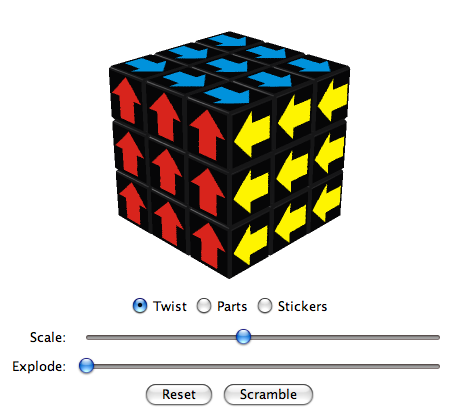# Virtual CubesActivate JavaScript to see the Virtual Cubes!

### Arrow Cube

A Super Cube is a design variation of the Rubik's Cube which visualizes the orientation of side parts. Because of this, a Super Cube has 2048 times more positions than a regular cube (46/2 = 2048).

Arrows on opposite faces are oriented in opposite directions as on a Shepherd's Cube.

The layout of the Arrow Cube was created in 2010 by Walter Randelshofer.

#### Algorithms to twist the centers

Algorithm
(U R U R')5 (20 ltm, 20 ftm, 20 qtm)
Permutation (++u)

Algorithm M. B. Thistlethwaite
(U · R L · U2 · R' L')2 (12* ltm, 12* ftm, 14 qtm)
Permutation (++u)

Algorithm
(U' R2 U2 R' U2 R2)3 (18 ltm, 18 ftm, 30 qtm)
Permutation (+u) (+r)

Algorithm Herbert Kociemba 2008
D' R · U D · R2 U2 MF MD B MD' MF' U2 R2 U' (14* ltm, 18 ftm, 22 qtm)
Permutation (+u) (+r)

Algorithm
(MR' MD' MR) U' (MR' MD MR) U (8* ltm, 14* ftm, 14* qtm)
Permutation (+u) (-r)

Algorithm
(MR' MD' MR) U2 (MR' MD MR) U2 (8* ltm, 14 ftm, 16* qtm)
Permutation (++u) (++r)

Algorithm
(MR' MD2 MR) U' (MR' MD2 MR) U (8* ltm, 14* ftm, 18* qtm)
Permutation (+u) (-d)

Algorithm
(MR' MD2 MR U2)2 (8* ltm, 14 ftm, 20 qtm)
Permutation (++u) (++d)

Algorithm Herbert Kociemba 2008
CD' · MR MD MR' D MR MF MR' F' MD MF' MD' F U' (13* ltm, 22 ftm, 22* qtm)
Permutation (+u) (+r) (+f) (+d) (-l) (+b)

Notation